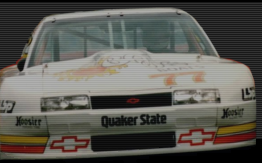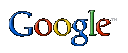BerettaFest 2021 is over - can't wait until next year!December 4, 2023
Information

Tire Calculation
Last Updated: June 22, 2003

205/55 R16.., what does this mean? Most people understand that the last number is the rim size in inches and the first number is the tread width in millimeters. But, what about the middle, number 55? Well, it is the aspect ratio, which indicates how tall the sidewall is. To find your sidewall height take the tread width and multiply it by this percentage and you get your sidewall height in mm. Percentages, metric and standard units of measurement, oh, the fun we are going to have here.

Now that you understand a few things about tire sizing. What can be done? Well, oodles really, including how many revolutions it makes in a mile (revs/mile), which can be used to calculate speed (revs/mile/time). To figure revolutions per mile we need to calculate only one thing, the overall tire height, which can be found by using the given information on the tire about its size. What gives a tire its overall tire height? Rim size and sidewall, of course. With the tire listed above we have a 16 in rim and a sidewall that is 112.75 mm tall (205 * 55%), but beings that the sidewall is at the top and bottom, multiplying that number by two gives, 225.5 mm of total sidewall height. To convert 225.5 mm to inches divide by the conversion 25.4 mm/in, which gives 8.88 in of total sidewall height. Accordingly, 16" + 8.88" = 24.88" of overall tire height.

So...

Rim size in inches + ((Tread width * aspect ratio * 2) / 25.4 mm/in) = Tire Height in inches

How are revolutions per mile calculated from overall tire height? Well if you remember from Geometry that the circumference of a circle is equal to the diameter (tire height) * pi, or 24.88" of overall tire height * pi = 78.16 in of circumference.

Now, one circumference is one revolution, so divide the circumference one mile...

63,360 inches per mile (conversion factor) / 78.16 in = 810.6 revolutions per mile

To find your revolutions per mile in one simple step, use the following combination of the above formulas.

63,360 (in per mile) /(((Tread Width (mm) * Aspect Ratio * 2)/25.4) + Rim Size * pi) = Revolutions per mileThe Web BerettaSpeed.com